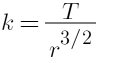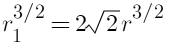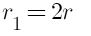SSC BOARD PAPERS IMPORTANT TOPICS COVERED FOR BOARD EXAM 2024

### Solve the following problems. If the height of a satellite completing one revolution around the earth in T seconds is h1 meter, then what would be the height of a satellite taking 2√2 T seconds for one revolution?

Question 11.

Solve the following problems.

If the height of a satellite completing one revolution around the earth in T seconds is h1 meter, then what would be the height of a satellite taking 2√2 T seconds for one revolution?

Given:
The height of the satellite completing one revolution around the earth in T seconds.

let the height be x of a satellite taking 2√2 T seconds for one revolution.

From Kelper’s third law of motion:

The square of the time period of revolution of the planet around the sun is directly proportional to the cube semi-major axis of its elliptical orbit.

T ∝ r3/2

Where
T is the time period
r is the radius of the satellite

Thus we can say,
T = K r3/2 ....(i)

Here, r1 = r when, T1 = t

We get,Then, if T2 = 2√2t

Putting the value in the equation(i), we get
2T = K r13/2
Putting the value of K in the above equation, we getOn solving the above equation, we getSquaring both side and then taking cube-root.....(ii)

Now r1= R + x(radius of earth + height above earth) and r=R+ h1
so using these relations we get
x=R + 2h1